# Protein Crystallography CourseCourse Homepage   Basic: 1 2 3 4 5 6   Advanced: 7 8 9 10 11 12 13 14 15 16 17 18 19 20 21 22

# Data collection

Andrew G.W. Leslie
MRC Laboratory of Molecular Biology, Hills Road, Cambridge UK

## Diffraction Geometry – The Reciprocal Lattice

An ideal crystal is composed of molecules arranged on a regular three dimensional lattice. The unit cell describes the basic building block for this lattice, and is characterised by the lengths of its three edges (a,b,c) and the angles between them (α,β,γ).

Definition: A lattice is an infinite arrangement of points in space where the environment of any point is identical to the environment of all other points.

This lattice (the real space lattice) is easily visualised in terms of the physical arrangement of molecules in the crystal. Indeed, it can often be seen directly by electron microscopy of a suitably thin crystal.

The reciprocal lattice is defined in the same way by the reciprocal unit cell, with axes a*, b*, c* (and interaxial angles α*,β*,γ*) by the following vector relationships: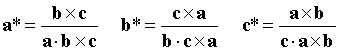Where "×" denotes a vector cross product and "·" denotes a vector dot product.

Thus a* is normal to the axes b and c, and has a magnitude inversely proportional to the magnitude of a.

In an orthogonal system (α=β=γ=90°) this simplifies to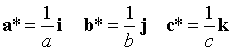where i, j k are unit vectors along the a, b, c axes.

The co-ordinates of the reciprocal lattice points are hkl where h, k and l are integers. Thus h is the number of reciprocal lattice points along the a* direction, k along b* and l along c*.

The reciprocal lattice has no obvious physical significance, but is extremely valuable in visualising diffraction geometry when used in conjunction with the Ewald sphere construction.

## The Ewald Sphere Construction

The Ewald sphere is centred on a line representing the X-ray beam direction, with a radius of 1/λ. The crystal position is at the centre of the Ewald sphere. The reciprocal lattice has its origin at the point where the X-ray beam exits the Ewald sphere. Rotation of the crystal (sitting at the centre of the sphere) results in an identical rotation of the reciprocal lattice about its origin. This is also discussed in Bernhard Rupp’s website on the Ewald sphere construction.

Diffraction from a set of planes with Miller indices hkl will occur when the corresponding reciprocal lattice point (hkl) lies exactly on the Ewald sphere. This is illustrated in an Ewald sphere animation.

(The way that the reciprocal lattice is defined means that the vector from the origin to the reciprocal lattice point hkl (d*) will be normal to the planes with Miller indices hkl and will have a magnitude 1/d, where d is the interplanar spacing. This is left as an exercise for the student.)

### Effects of crystal mosaicity, wavelength dispersion and beam divergence

In practice, because a real crystal is made up of many small mosaic blocks with a small spread in orientations, a reciprocal lattice point for the crystal will not be a true point, but a small spherical cap. In the extreme case of a powder, each reciprocal lattice point becomes a spherical shell.

Equally, because of wavelength dispersion, the Ewald sphere has a finite thickness. Beam divergence will also increase the effective thickness of the Ewald sphere.

As a consequence, particularly for macromolecular crystals which have relatively large unit cells (and thus closely spaced reciprocal lattice points), a number of planes will be in a diffracting position even for a stationary crystal.

However, in order to bring all planes into a diffracting condition (in a monochromatic experiment) the crystal must be rotated. As the crystal rotates, the reciprocal lattice also rotates about its own origin, and a succession of reciprocal lattice points will pass through the Ewald sphere.

### The Ewald sphere construction explains the appearance of diffraction patterns

If the X-ray beam is along a principal zone axis direction, it will be normal to a set of densely populated reciprocal lattice planes. These planes will intersect the Ewald sphere in a set of concentric circles, centred on the direct beam position.

Thus all reciprocal lattice points in a diffracting condition will also lie on this set of concentric circles. The distance between the circles is a function of the spacing of the reciprocal lattice planes along the X-ray beam direction.

If the crystal is rotated through a small angle, each of these circles will be drawn out into a lune. Within the lune, the spot separation will be determined by the reciprocal lattice spacings within the planes.

A very mosaic crystal (or a large beam divergence) has an effect on the diffraction pattern that is very similar to rotation of the crystal. Instead of circles of spots on a "still" image (no crystal rotation), lunes of spots will be seen.

If a principal zone axis is precessed about the X-ray beam direction, with the detector following the same precessive motion, then an undistorted projection of the reciprocal lattice can be recorded (a precession photograph) from which the lattice parameters can be measured directly.

## Symmetry of the reciprocal lattice

The symmetry of the reciprocal lattice follows the symmetry of the real space lattice. This symmetry extends beyond the geometric arrangement of the reciprocal lattice points themselves, and includes the diffracted intensities associated with each r.l.p. In addition, in the absence of anomalous scattering, the diffracted intensities obey Friedel’s Law:

I(hkl) = I(-h,-k,-l)

Thus the reciprocal lattice has a centre of symmetry (which in general is not true for the real space lattice).

In the absence of crystal symmetry, a 180° rotation of the crystal is required to measure (nearly…see below) all the unique data. Because of Friedel’s law, this will result in a multiplicity of two. However, in the presence of symmetry, a data collection strategy can be employed which requires a smaller rotation to measure all the unique data. For example, if the crystal belongs to the Laue group 422, and is rotated around the four-fold axis during data collection, then only a 45° rotation is required (but it must be the correct 45°). A monoclinic crystal requires a 180° rotation if rotated around the unique b axis, but only 90° if rotated around the a or c axes.

The required rotation depends on both the crystal orientation and the Laue group. Programs are available to help design a suitable strategy.

## The "cusp" data

Reciprocal lattice points lying very close to the rotation axis will never pass through the Ewald sphere. If the crystal is aligned with its symmetry axis exactly along the rotation axis, or if there is no symmetry (spacegroup P1), these data will not be recorded even if a full 360° rotation is used. To record these "cusp" data, the crystal must be rotated around a second axis (preferably orthogonal to the first). This is always necessary for triclinic data. For other symmetries, the missing data can be avoided by offsetting the symmetry axis from the rotation axis; in this case a reflection that lies in the cusp volume will have a symmetry mate that will pass through the Ewald sphere. The volume of the cusp region depends on the resolution and the wavelength. For wavelengths of 1Å or less, and 2.5Å resolution (or lower), the percentage of data that is lost is negligably small.

## Data Collection

Although in principle there are many ways in which the required volume of reciprocal space can be covered (e.g. precession photography), in practice this is always achieved by a simple rotation of the crystal about a single axis (the rotation or oscillation method).

In some experimental designs the crystal is placed on a multiple axis goniostat, allowing it to be oriented in a particular way (for example, with a symmetry axis parallel to the rotation axis). In the majority of cases, however, a single axis goniostat is all that is required.

As discussed earlier, the crystal orientation and crystal symmetry (Laue group) determine the total rotation range required. This range is covered by a series of sequential rotations. There are several factors which influence the choice of oscillation (rotation) angle for each image. A large oscillation angle minimises the number of images that need to be collected, and therefore the "dead time" while each image is read out and written to disk. However the angle must not be so large that spots on adjacent lunes overlap on the detector. A large oscillation angle also has the disadvantage that it increases the X-ray background on the image, which degrades the signal to noise, especially for the weak, high resolution data.

Coarse phi-slicing corresponds to the situation when the oscillation angle is comparable to or greater than the width (in phi) of a typical reflection. If the image width is a fraction of the reflection width, this is referred to as fine phi-slicing.

### Data Processing

Data processing falls naturally into three quite distinct steps:

i. Determination of crystal cell parameters, space group and orientation.

ii. Integration of the images (with concurrent refinement of crystal, beam and detector parameters).

iii. Data reduction, that is placing all data on a common scale, merging multiple observations to give a unique dataset while rejecting outliers and reducing intensities to amplitudes for use in heavy atom phasing, Fourier syntheses, model refinement etc.

### Autoindexing

Autoindexing uses the positions of spots on one of more images to determine the crystal lattice parameters and its orientation. The most successful algorithms are based on an FFT approach.

It is important to realise that it is NOT possible to determine the space group symmetry from the autoindexing alone, as it only makes use of spot positions. Spot intensities are required to detect the presence of symmetry.

### Definition of crystal orientation

The crystal orientation can be defined as

X  =  Φ U B h

where

X is a vector in the laboratory frame giving the position of the reciprocal lattice vector with indices h

B is an orthogonalisation matrix, which  defines a set of orthogonal axes based on the crystal axes. This matrix depends only on the crystal cell parameters.

U is a pure rotation matrix describing the orientation of the crystal in the laboratory frame in the initial or standard setting.

Φ is the rotation around the spindle axis for a single axis device, or more generally the goniostat matrix.

For convenience, the product of the U and B matrices is often denoted as the "setting matrix" A

A U B

The orthogonalisation matrix B in the general case is given by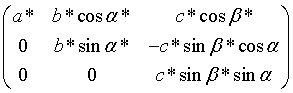which as mentioned earlier depends only on the crystal cell parameters.

### Parameter refinement

Once an orientation matrix and cell parameters have been derived from the auto-indexing, these parameters (and others) are refined further using a different algorithm.  The parameters to be refined can be conveniently grouped into three classes:

a) Crystal parameters:  cell parameters, crystal orientation and mosaic spread (isotropic or anisotropic).

b) Detector parameters:  the detector position and orientation and (if appropriate) distortion parameters (e.g. the radial and tangential offsets for the Mar image plate scanner).

c) Beam parameters:  the orientation of the primary beam and beam divergence (isotropic or anisotropic).

The refinement of these parameters is achieved by least-squares minimisation of two residuals; a positional residual: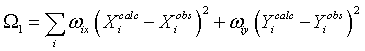where X and Y are the spot co-ordinates on the detector, and an angular residual: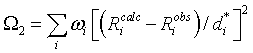where Ricalc, Riobs are the calculated and observed distances of the reciprocal lattice point di* from the centre of the Ewald sphere, shown in the figure below.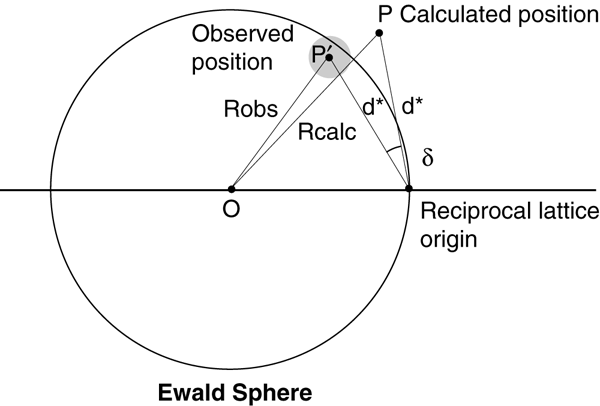The reciprocal lattice point is represented as a gray sphere at position P', which lies at a distance Robs from the centre of the Ewald sphere. The point P represents the calculated position of the reciprocal lattice point.

Riobs is obtained from the phi centroid if fine phi slices have been used.  For coarse phi slices, the reciprocal lattice point is either assumed to lie exactly on the Ewald sphere at the midpoint of the rotation, or for partially recorded reflections its position is estimated from the degree of partiality of the reflection (i.e. the way in which the total intensity is distributed between the two abutting images).  This latter approach, known as post-refinement because it requires a knowledge of the integrated intensities, requires a model for the rocking curve, and permits refinement of either crystal mosaicity or beam divergence. For fine phi slices the mosaic spread or beam divergence is estimated from the observed reflection width in phi.

The refinement strategy can depend on how the data has been collected.  If fine phi slices have been used, accurate phi centroids and co-ordinates (X,Y) are available for most strong reflections (excluding those very close to the rotation axis) and both residuals (Ω1, 2) can be minimised simultaneously using a suitable selection of reflections (strong and evenly distributed over the detector and in phi).  Problems arising due to correlations of different parameters can be avoided either by fixing some parameters or by the use of eigen-value filtering.  These problems can be particularly serious for low resolution data, where there is a strong correlation between crystal to detector distance and the cell parameters, or for an offset detector where there is a high correlation between the detector swing angle and the (horizontal) primary beam co-ordinate.  If only a narrow phi range of reflections is used in the refinement then some unit cell parameters will be poorly defined and may be correlated with the crystal setting angles, and there will also be a strong correlation between the detector orientation around the X-ray beam and the crystal setting angle around the beam.  In such circumstances the refined parameters may assume physically unrealistic values, but this will not necessarily impair the accuracy of the prediction of reflection positions and widths.

When the data is collected with coarse phi slices, only fully recorded reflections will give accurate spot positions (X,Y), and accurate phi centroids can only be determined for partially recorded reflections.  In some the two residuals are therefore minimised independently.  Only the detector parameters are refined when minimising the positional residual, and only cell orientation and optionally beam parameters are refined against the angular residual.  This approach does have the advantage that the accuracy of the refined cell parameters does not depend on the accuracy of the crystal to detector distance or direct beam position, providing these are known sufficiently well to allow correct indexing of the reflections.

### Integration of the Images

Predict the position in the digitised image of each Bragg reflection.

Estimating its intensity (after subtracting the X-ray background) and an error estimate of the intensity.

### Predicting reflection positions

Prediction of spot positions on a "virtual detector"

Map onto the digitised image allowing for spatial distortions.

The background can only be measured in a region around the spot either in two dimensions (X, Y, the detector co-ordinates) for coarse phi-slices or in 3 dimensions (X, Y and phi) for fine phi slices.

Require definition of a peak/background mask.

Errors in the mask definition will give systematic errors in intensities.

### Summation integration and Profile Fitting

#### Summation integration

Sum the pixel values of all pixels in the peak area of the mask, and then subtract the sum of the background values calculated from the background plane for the same pixels.

#### Profile fitting

Assume that the shape or profile (in 2 or 3 dimensions) of the spots is known.

Determine the scale factor which, when applied to the known spot profile, gives the best fit to be observed spot profile.  This scale factor is then proportional to the profile fitted intensity for the reflection.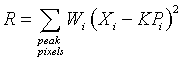Xi     is the background subtracted intensity at pixel i

Pi     is the value of the standard profile at the corresponding pixel

Wi      is a weight, derived from the expected variance of Xi

K     is the scale factor to be determined

#### Determining the "Standard" Profile

These are determined for different areas on the detector. A weighted mean is used to evaluate any individual reflection.

Precautions must be taken to avoid introducing systematic errors because of errors in the "standard" profiles.

### Standard Deviation Estimates

For summation integration, a standard deviation can be obtained based on Poisson statistics.

For profile fitted intensities the goodness of fit of the scaled standard profile to the true reflection profile can be used.

These will generally underestimate the true errors, and should be modified accordingly.

### Scaling

Apply polarisation and Lorentz factor corrections.

The intensities from different images then need to be put on a common scale. This allows for variations in source intensity (e.g. beam decay at a synchrotron), variations in diffracting volume, radiation damage and, to some extent, absorption corrections.

Apply a scale factor K and temperature factor B to each image, and these parameters are refined to minimise the residual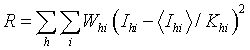where:

Ihi is the ith measurement of reflection h

whi is the weight for that observation (the inverse of the variance)

<Ihi> is the weighted mean intensity for reflection h

Khi   =   Kj exp (-2Bjsin2θh2)

Kj and Bj are the scale and temperature factors for image j on which Ihi was measured.

θh is the Bragg angle for reflection h

Success depends on the presence of multiple (symmetry related) observations on different images.

### Merging data

Multiple observations are reduced to a weighted mean intensity and standard deviation.

"Rogue" observations or outliers detected and rejected.

Statistics on the agreement between multiple observations, data completeness, evidence for systematic errors such as partial bias (which arise from errors in modelling the reflection width) are also calculated at this stage.

#### Modifying Standard Deviations.

The level of agreement between multiple observations can be used to modify the standard deviations of the intensities.  Providing the multiplicity is high, then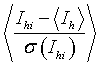where:

Ihi, σ(Ihi) are the intensity and standard deviation of the ith observation of reflection h

<Ih> is the weighted mean intensity

should equal unity when averaged over a significant number of reflections.

Standard deviations are modified to give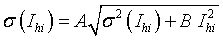where the values of the parameters A and B are chosen to get a standard deviation ratio of unity for all intensity ranges.

#### Reducing Intensities to Amplitudes

The simplest way to determine the structure factor amplitudes (F) from the observed intensities (I) is simply

F = √I

However this cannot be applied to observations for which the observed intensity is negative (statistically, a certain percentage of the data will be expected to have negative intensities).  To overcome this problem, French and Wilson have applied Bayesian statistics, making use of the prior knowledge that  the true intensity must be greater than zero, and the distribution of intensities obeys Wilson statistics, to derive the most likely (positive) intensity for those reflections with an observed negative intensity.